Note: The other languages of the website are Google-translated. Back to English

## How to quickly convert numbers to English words in Excel?

If you have a column of numeric values in a worksheet, and now you need to convent them to their equivalent English words or English currency words as following screenshot shown. Here I will talk about how to change the numbers to English words quickly and easily.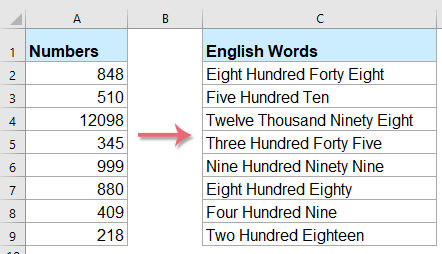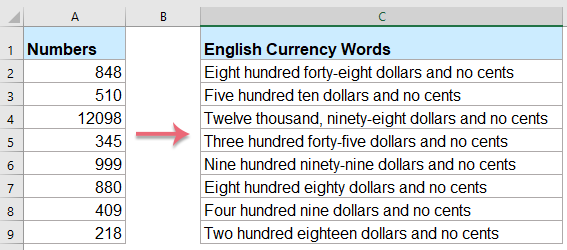Convert numbers to English words with User Defined Function

Convert numbers to currency words with User Defined Function

Convert numbers to currency words with a useful feature

#### Convert numbers to English words with User Defined Function

There are no features or formulas can help you to solve this task directly in Excel, but you can create a User Defined Function to finish it. Please do with following steps:

1. Hold down the ALT + F11 keys, and it opens the Microsoft Visual Basic for Applications Window.

2. Click Insert > Module, and paste the following code in the Module Window.

``````Function NumberstoWords(ByVal MyNumber)
'Update by Extendoffice
Dim xStr As String
Dim xFNum As Integer
Dim xStrPoint
Dim xStrNumber
Dim xPoint As String
Dim xNumber As String
Dim xP() As Variant
Dim xDP
Dim xCnt As Integer
Dim xResult, xT As String
Dim xLen As Integer
On Error Resume Next
xP = Array("", "Thousand ", "Million ", "Billion ", "Trillion ", " ", " ", " ", " ")
xNumber = Trim(Str(MyNumber))
xDP = InStr(xNumber, ".")
xPoint = ""
xStrNumber = ""
If xDP > 0 Then
xPoint = " point "
xStr = Mid(xNumber, xDP + 1)
xStrPoint = Left(xStr, Len(xNumber) - xDP)
For xFNum = 1 To Len(xStrPoint)
xStr = Mid(xStrPoint, xFNum, 1)
xPoint = xPoint & GetDigits(xStr) & " "
Next xFNum
xNumber = Trim(Left(xNumber, xDP - 1))
End If
xCnt = 0
xResult = ""
xT = ""
xLen = 0
xLen = Int(Len(Str(xNumber)) / 3)
If (Len(Str(xNumber)) Mod 3) = 0 Then xLen = xLen - 1
Do While xNumber <> ""
If xLen = xCnt Then
xT = GetHundredsDigits(Right(xNumber, 3), False)
Else
If xCnt = 0 Then
xT = GetHundredsDigits(Right(xNumber, 3), True)
Else
xT = GetHundredsDigits(Right(xNumber, 3), False)
End If
End If
If xT <> "" Then
xResult = xT & xP(xCnt) & xResult
End If
If Len(xNumber) > 3 Then
xNumber = Left(xNumber, Len(xNumber) - 3)
Else
xNumber = ""
End If
xCnt = xCnt + 1
Loop
xResult = xResult & xPoint
NumberstoWords = xResult
End Function
Function GetHundredsDigits(xHDgt, xB As Boolean)
Dim xRStr As String
Dim xStrNum As String
Dim xStr As String
Dim xI As Integer
Dim xBB As Boolean
xStrNum = xHDgt
xRStr = ""
On Error Resume Next
xBB = True
If Val(xStrNum) = 0 Then Exit Function
xStrNum = Right("000" & xStrNum, 3)
xStr = Mid(xStrNum, 1, 1)
If xStr <> "0" Then
xRStr = GetDigits(Mid(xStrNum, 1, 1)) & "Hundred "
Else
If xB Then
xRStr = "and "
xBB = False
Else
xRStr = " "
xBB = False
End If
End If
If Mid(xStrNum, 2, 2) <> "00" Then
xRStr = xRStr & GetTenDigits(Mid(xStrNum, 2, 2), xBB)
End If
GetHundredsDigits = xRStr
End Function
Function GetTenDigits(xTDgt, xB As Boolean)
Dim xStr As String
Dim xI As Integer
Dim xArr_1() As Variant
Dim xArr_2() As Variant
Dim xT As Boolean
xArr_1 = Array("Ten ", "Eleven ", "Twelve ", "Thirteen ", "Fourteen ", "Fifteen ", "Sixteen ", "Seventeen ", "Eighteen ", "Nineteen ")
xArr_2 = Array("", "", "Twenty ", "Thirty ", "Forty ", "Fifty ", "Sixty ", "Seventy ", "Eighty ", "Ninety ")
xStr = ""
xT = True
On Error Resume Next
If Val(Left(xTDgt, 1)) = 1 Then
xI = Val(Right(xTDgt, 1))
If xB Then xStr = "and "
xStr = xStr & xArr_1(xI)
Else
xI = Val(Left(xTDgt, 1))
If Val(Left(xTDgt, 1)) > 1 Then
If xB Then xStr = "and "
xStr = xStr & xArr_2(Val(Left(xTDgt, 1)))
xT = False
End If
If xStr = "" Then
If xB Then
xStr = "and "
End If
End If
If Right(xTDgt, 1) <> "0" Then
xStr = xStr & GetDigits(Right(xTDgt, 1))
End If
End If
GetTenDigits = xStr
End Function
Function GetDigits(xDgt)
Dim xStr As String
Dim xArr_1() As Variant
xArr_1 = Array("Zero ", "One ", "Two ", "Three ", "Four ", "Five ", "Six ", "Seven ", "Eight ", "Nine ")
xStr = ""
On Error Resume Next
xStr = xArr_1(Val(xDgt))
GetDigits = xStr
End Function``````

3. Save and close this code and go back the worksheet, in a blank cell, enter this formula =NumberstoWords(A2)( A2 is the cell you want to convert the number to an English word), see screenshot: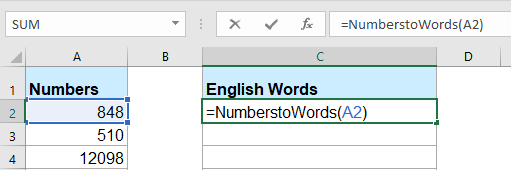4. Then press Enter key, and select cell C2 then drag the fill handle to the range that you want to contain this formula. All the numeric values have been converted their corresponding English words.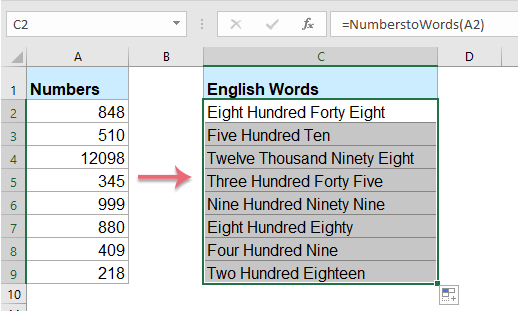Note: This code only works for integer numbers but does not apply to decimal numbers.

#### Convert numbers to currency words with User Defined Function

If you want to convert the numbers into English currency words, you should apply the below VBA code.

1. Hold down the ALT + F11 keys, and it opens the Microsoft Visual Basic for Applications Window.

2. Click Insert > Module, and paste the following code in the Module Window.

``````Function SpellNumberToEnglish(ByVal pNumber)
'Update by Extendoffice
Dim Dollars, Cents
arr = Array("", "", " Thousand ", " Million ", " Billion ", " Trillion ")
pNumber = Trim(Str(pNumber))
xDecimal = InStr(pNumber, ".")
If xDecimal > 0 Then
Cents = GetTens(Left(Mid(pNumber, xDecimal + 1) & "00", 2))
pNumber = Trim(Left(pNumber, xDecimal - 1))
End If
xIndex = 1
Do While pNumber <> ""
xHundred = ""
xValue = Right(pNumber, 3)
If Val(xValue) <> 0 Then
xValue = Right("000" & xValue, 3)
If Mid(xValue, 1, 1) <> "0" Then
xHundred = GetDigit(Mid(xValue, 1, 1)) & " Hundred "
End If
If Mid(xValue, 2, 1) <> "0" Then
xHundred = xHundred & GetTens(Mid(xValue, 2))
Else
xHundred = xHundred & GetDigit(Mid(xValue, 3))
End If
End If
If xHundred <> "" Then
Dollars = xHundred & arr(xIndex) & Dollars
End If
If Len(pNumber) > 3 Then
pNumber = Left(pNumber, Len(pNumber) - 3)
Else
pNumber = ""
End If
xIndex = xIndex + 1
Loop
Select Case Dollars
Case ""
Dollars = "No Dollars"
Case "One"
Dollars = "One Dollar"
Case Else
Dollars = Dollars & " Dollars"
End Select
Select Case Cents
Case ""
Cents = " and No Cents"
Case "One"
Cents = " and One Cent"
Case Else
Cents = " and " & Cents & " Cents"
End Select
SpellNumberToEnglish = Dollars & Cents
End Function
Function GetTens(pTens)
Dim Result As String
Result = ""
If Val(Left(pTens, 1)) = 1 Then
Select Case Val(pTens)
Case 10: Result = "Ten"
Case 11: Result = "Eleven"
Case 12: Result = "Twelve"
Case 13: Result = "Thirteen"
Case 14: Result = "Fourteen"
Case 15: Result = "Fifteen"
Case 16: Result = "Sixteen"
Case 17: Result = "Seventeen"
Case 18: Result = "Eighteen"
Case 19: Result = "Nineteen"
Case Else
End Select
Else
Select Case Val(Left(pTens, 1))
Case 2: Result = "Twenty "
Case 3: Result = "Thirty "
Case 4: Result = "Forty "
Case 5: Result = "Fifty "
Case 6: Result = "Sixty "
Case 7: Result = "Seventy "
Case 8: Result = "Eighty "
Case 9: Result = "Ninety "
Case Else
End Select
Result = Result & GetDigit(Right(pTens, 1))
End If
GetTens = Result
End Function
Function GetDigit(pDigit)
Select Case Val(pDigit)
Case 1: GetDigit = "One"
Case 2: GetDigit = "Two"
Case 3: GetDigit = "Three"
Case 4: GetDigit = "Four"
Case 5: GetDigit = "Five"
Case 6: GetDigit = "Six"
Case 7: GetDigit = "Seven"
Case 8: GetDigit = "Eight"
Case 9: GetDigit = "Nine"
Case Else: GetDigit = ""
End Select
End Function``````

3. Save this code and go back the worksheet, in a blank cell, enter this formula =SpellNumberToEnglish(A2)( A2 is the cell you want to convert the number to an English currency word), and then drag the fill handle down to the cells that you want to apply this formula, all the numbers have been spelt out into English currency words, see screenshot: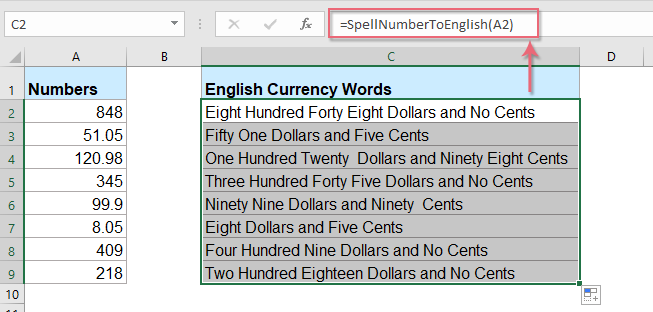#### Convert numbers to currency words with a useful feature

The above code is somewhat troublesome for Excel beginners, here, I will introduce a useful feature, Kutools for Excel's Numbers to Words, with this utility, you can quickly spell out the numbers into English words or currency words.

Tips:To apply this Numbers to Words feature, firstly, you should download the Kutools for Excel, and then apply the feature quickly and easily.

After installing Kutools for Excel, please do as this:

1. Select the list of numbers you want to convert, and then click Kutools > Content > Numbers to Words, see screenshot: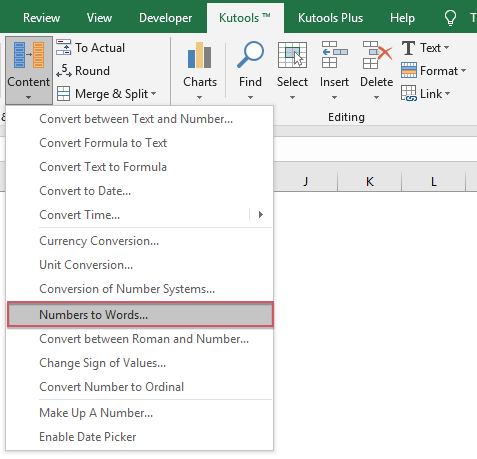2. In the Numbers to Currency Words dialoge box, check English option from the Languages list box, and then click Ok button, you will see all the numbers have been spelt out into English currency words: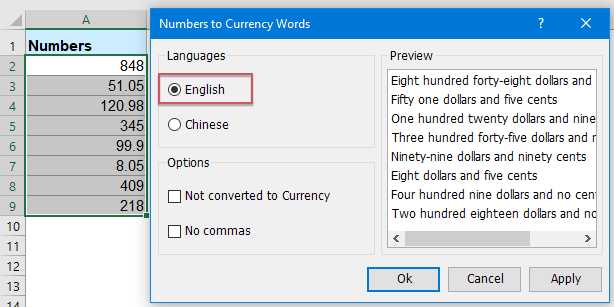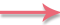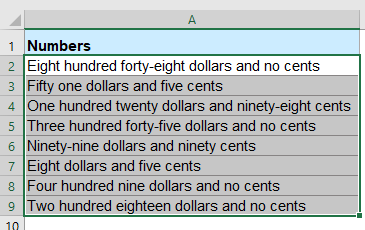Tips: If you want to get the English words, please check the Not converted to Currency check box from the Options section, see screenshots: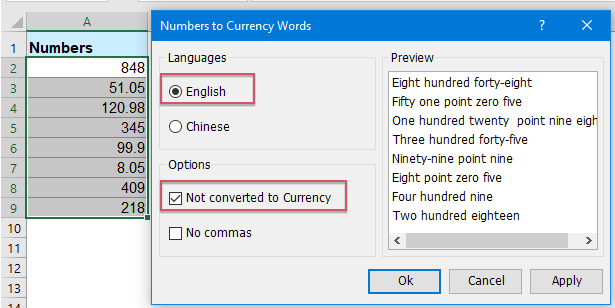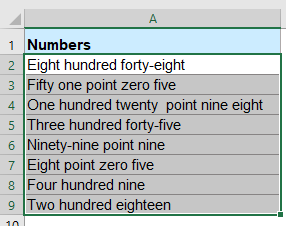### The Best Office Productivity Tools

#### Kutools for Excel Solves Most of Your Problems, and Increases Your Productivity by 80%

• Reuse: Quickly insert complex formulas, charts and anything that you have used before; Encrypt Cells with password; Create Mailing List and send emails...
• Super Formula Bar (easily edit multiple lines of text and formula); Reading Layout (easily read and edit large numbers of cells); Paste to Filtered Range...
• Merge Cells/Rows/Columns without losing Data; Split Cells Content; Combine Duplicate Rows/Columns... Prevent Duplicate Cells; Compare Ranges...
• Select Duplicate or Unique Rows; Select Blank Rows (all cells are empty); Super Find and Fuzzy Find in Many Workbooks; Random Select...
• Exact Copy Multiple Cells without changing formula reference; Auto Create References to Multiple Sheets; Insert Bullets, Check Boxes and more...
• Extract Text, Add Text, Remove by Position, Remove Space; Create and Print Paging Subtotals; Convert Between Cells Content and Comments...
• Super Filter (save and apply filter schemes to other sheets); Advanced Sort by month/week/day, frequency and more; Special Filter by bold, italic...
• Combine Workbooks and WorkSheets; Merge Tables based on key columns; Split Data into Multiple Sheets; Batch Convert xls, xlsx and PDF...
• More than 300 powerful features. Supports Office/Excel 2007-2019 and 365. Supports all languages. Easy deploying in your enterprise or organization. Full features 30-day free trial. 60-day money back guarantee.#### Office Tab Brings Tabbed interface to Office, and Make Your Work Much Easier

• Enable tabbed editing and reading in Word, Excel, PowerPoint, Publisher, Access, Visio and Project.
• Open and create multiple documents in new tabs of the same window, rather than in new windows.
• Increases your productivity by 50%, and reduces hundreds of mouse clicks for you every day!No ratings yet. Be the first to rate!
This comment was minimized by the moderator on the site
Hi.. Good morningHi.. Good morning.. It is nice to meet you.
This comment was minimized by the moderator on the site
this code is not working
This comment was minimized by the moderator on the site
Sir how can i convert numerical to words with last word "Only" ?
This comment was minimized by the moderator on the site
problem solve?????
This comment was minimized by the moderator on the site
="Rs."&""&F6&"Only"&"."
This comment was minimized by the moderator on the site
This comment was minimized by the moderator on the site
Thanks for your kind support for creating formula. Can you please tell us if we need to add the name of currency..like Saudi Riyals or British Pounds.
This comment was minimized by the moderator on the site
For example we convert 4000 into word "Four Thousand", I want to translate it like " Saudi Riyals Four Thousand Only" so missing in formula is "Saudi Riyals & Only"
This comment was minimized by the moderator on the site
Above mentioned formula in module is repeated and copied from Microsoft Website..

https://support.microsoft.com/en-in/help/213360/how-to-convert-a-numeric-value-into-english-words-in-excel
This comment was minimized by the moderator on the site
dear this code is showing syntax error in this line
Cents = GetTens(Left(Mid(MyNumber, DecimalPlace + 1) &_"00", 2))

can you help me ..??Reply me AS soon As possible
This comment was minimized by the moderator on the site
ADD THE WORD AND BETWEEN WORDS. One Hundred and Forty Thousand, Five Hundred and Twenty Six instead of One Hundred Forty Thousand Five Hundred Twenty Six. The sentence may not be understood well as its not proper english.
This comment was minimized by the moderator on the site
this code is not working permenatly ...if we close and open the sheet its not working
This comment was minimized by the moderator on the site
Save the file as "Macro Enabled Work Book" and enable Macros when you open it
This comment was minimized by the moderator on the site
thanks for lot for u are code
This comment was minimized by the moderator on the site
For example we convert 2500 into word "Two Thousand Five Hundred", I want to translate it like " Two Thousand Five Hundred Only" so missing in formula is "Only"
This comment was minimized by the moderator on the site
use =CONCATENATE(NumberstoWords(A1)," Only")
This comment was minimized by the moderator on the site
Really thanks dear, your formula was perfect, good, Allah Bless you.
Regards.
This comment was minimized by the moderator on the site
lakh crore not in the formula. Kindly help me.
This comment was minimized by the moderator on the site
For example we convert 2500 into word "Two Thousand Five Hundred", I want to translate it like " Two Thousand Five Hundred & Cents Fifty Only" so could you please help
This comment was minimized by the moderator on the site
I'm not getting the cents spell out... my amount is 123.50 and got "one hundred twenty three"... copied all the formula, please help
This comment was minimized by the moderator on the site
This comment was minimized by the moderator on the site
Thanks a lot
This comment was minimized by the moderator on the site
FINE. But sorry to say that this formula is NOT working permanently ... I closed the workbook for Lunch break. After Lunch I opened the file but the formula was not working. showing error like #NAME?. Kindly intimate solutions please.
This comment was minimized by the moderator on the site
Thanks

Its working! :)
This comment was minimized by the moderator on the site
there is a bug when my amount is "618475" it says "Six Hundred Eighteen Thousand Four Hundred Seventy Five" instead of "six lac eighteen thousand four hundred seventy five".
This comment was minimized by the moderator on the site
I have thesame problem
This comment was minimized by the moderator on the site
This is not a problem, it is a format of writing in words
This comment was minimized by the moderator on the site
Thank you extendOfficea
There are no comments posted here yet